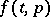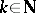# Quotient space

(diff) ← Older revision | Latest revision (diff) | Newer revision → (diff)

of a dynamical systemgiven on a topological spaceThe quotient space ofrelative to the equivalence:if the pointsandbelong to the same trajectory. In other words, the points of the quotient space are the trajectories of the dynamical system(in a different notation, see ), and the topology is the strongest in which the mapping associating each point ofwith its trajectory is continuous (thus,(is a directed set) if and only if there aresuch thatifis a metric space, then). The quotient spaces of many dynamical systems do not satisfy any of the separation axioms, even ifdoes. For example, ifis a minimal set, then the closure of every non-empty set in the quotient space is the whole quotient space. If a dynamical system given on a metric space is completely unstable (see Complete instability), then for its quotient space to be Hausdorff it is necessary and sufficient that this dynamical system does not have saddles at infinity (cf. Saddle at infinity).

How to Cite This Entry:
Quotient space. Encyclopedia of Mathematics. URL: http://encyclopediaofmath.org/index.php?title=Quotient_space&oldid=11882
This article was adapted from an original article by V.M. Millionshchikov (originator), which appeared in Encyclopedia of Mathematics - ISBN 1402006098. See original article